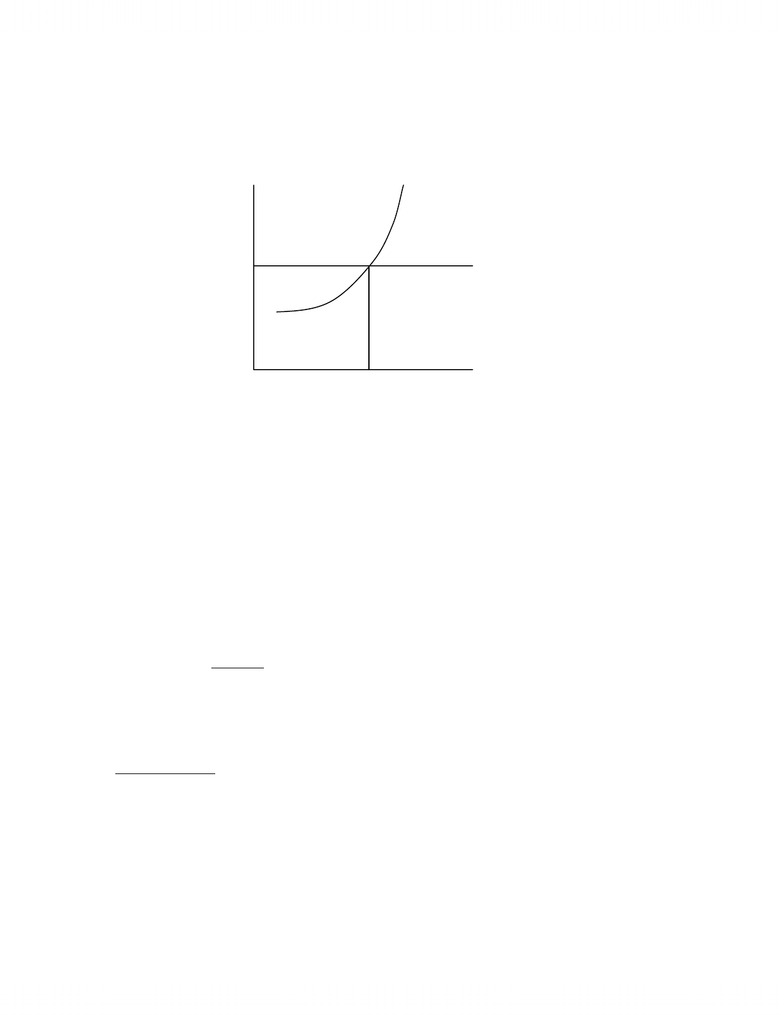# Lecture 15-Perfect Competition pt.2

24 views4 pages
School
Department
Course
ProfessorThursday, November 5th, 2009.
Perfect Competition
MR = MC => q* is profit-maximizing level of output
Question: Is firm earning economic profits?
Is firm breaking even (zero economic profits)?
Is firm suffering economic loss?
Economic Profit = Total Revenue
Minus
Total Opportunity Cost (Explicit + Implicit)
Most Important Implicit Opportunity Cost: Invested Capital (Equity)
,QYHVWHG&DSLWDOHDUQV³QRUPDOUDWHRISURILW´Æ Economic Profit = Zero
(and Accounting Profit is Positive)
Level of profits:
1. Firm maximizes profits by producing where P = MC
2. Levels of profits:
TR > TC => profits => TR/Q > TC/Q Ù P > ATC
TR = TC => breakeven Ù P = ATC
TR < TC => loss => TR/Q < TC/Q Ù P < ATC
P
MR [= P]
q*
MC
q
www.notesolution.com
Unlock document

This preview shows page 1 of the document.
Unlock all 4 pages and 3 million more documents.

## Document Summary

Mr = mc => q* is profit-maximizing level of output. To answer: add atc schedule compare p to atc. Most important implicit opportunity cost: invested capital (equity) 3;0890/,59,0,7383472,7,904157419 economic profit = zero (and accounting profit is positive) Level of profits: firm maximizes profits by producing where p = mc, levels of profits: Tr > tc => profits => tr/q > tc/q p > atc. Tr = tc => breakeven p = atc. Tr < tc => loss => tr/q < tc/q p < atc www. notesolution. com. Profit: (p atc) * q = (25 20) * 10 = 50. Economic profit: (p atc) * q = (25 30) * 10 = -50. Decisions of perfectly competitive firm: summary: choose output (q) that maximizes profit. P = mc: determine if should shut down in short-run* P < avc shut down (otherwise, continue to produce q) www. notesolution. com: determine whether to exit industry in long-run.

## Get access

\$10 USD/m
Billed \$120 USD annuallyHomework Help
Study Guides
Textbook Solutions
Class Notes
Textbook Notes
Booster Class